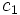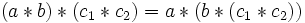# Right-associative elements of magma form submagma

## Statement

Let$(S,*)$ be a magma (a set$S$ with binary operation$*$). Call an element$c \in S$ a right-associative element if the following holds:$a * (b * c) = (a * b) * c \ \forall \ a,b \in S$

Then:

1. The set of right-associative elements of$S$ forms a submagma of$S$. This submagma is termed the Right nucleus (?) of the magma.
2. If$S$ contains a right neutral element$e$, then$e$ is right-associative, and is a right neutral element for the submagma of right-associative elements.
3. If$S$ contains a right nil$n$, then$n$ is right-associative, and is a right nil for the submagma of right-associative elements.

## Related facts

All these proofs make crucial use of the associativity pentagon: the pentagon describing the relation between the five different ways of associating a product of length four.

## Proof

### Proof idea

The idea behind the proof is the associativity pentagon, which states that the five different ways of parenthesizing an expression of length four form a cyclic pentagon, with each expression related to exactly two others via a single application of the associativity law. In order to move along one edge of the pentagon, we instead move along the path comprising the remaining four edges, and use the fact that each step there is allowed because one of$c_1$ and$c_2$ is the right term in each of the applications of the law.

### Proof of (1)

Given: A magma$(S,*)$, two right-associative (i.e., right nuclear) elements$c_1,c_2 \in S$

To prove:$c_1 * c_2$ is right-associative (i.e., right nuclear)

Proof: We need to show that, for any$a, b \in S$, we have:$(a * b) * (c_1 * c_2) = a * (b * (c_1 * c_2))$.

Let's do this. Start with the left side and proceed as follows:$(a * b) * (c_1 * c_2) = ((a * b) * c_1) * c_2 = (a * (b * c_1)) * c_2 = a * ((b * c_1) * c_2) = a * (b * (c_1 * c_2))$.

Here is a diagrammatic depiction of the proof:

### Proof of (2)

Given: A magma$(S,*)$ with right neutral element$e$.

To prove:$e$ is in the submagma of right-associative elements and is right neutral for the submagma.

Proof: For any$a,b \in S$,$(a * b) * e = a * b = a * (b * e)$, so$e$ is right-associative. Since$b * e = b$ for all$b \in S$,$b * e = b$ for all right-associative elements in particular.

### Proof of (3)

Given: A magma$(S,*)$ with right nil$n$.

To prove:$n$ is in the submagma of right-associative elements and is a right nil for that submagma.

Proof: For any$a,b \in S$,$(a * b) * n = n = b * n = (a * b) * n$, so$n$ is right-associative. Since$b * n = n$ for all$b \in S$,$b * n = n$ for all right-associative elements in particular.